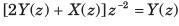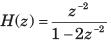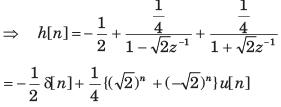Courses

# Test: Z-Transform Part - 3

## 10 Questions MCQ Test Signal and System | Test: Z-Transform Part - 3

Description
This mock test of Test: Z-Transform Part - 3 for Electrical Engineering (EE) helps you for every Electrical Engineering (EE) entrance exam. This contains 10 Multiple Choice Questions for Electrical Engineering (EE) Test: Z-Transform Part - 3 (mcq) to study with solutions a complete question bank. The solved questions answers in this Test: Z-Transform Part - 3 quiz give you a good mix of easy questions and tough questions. Electrical Engineering (EE) students definitely take this Test: Z-Transform Part - 3 exercise for a better result in the exam. You can find other Test: Z-Transform Part - 3 extra questions, long questions & short questions for Electrical Engineering (EE) on EduRev as well by searching above.
QUESTION: 1

### The z-transform of a signal x[n] is given by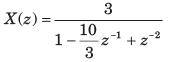If X (z) converges on the unit circle, x[n] is

Solution: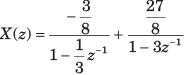Since X (z) converges on |z|= 1. So ROC must include this circle.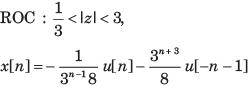QUESTION: 2

### The transfer function of a system is given as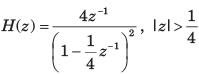The h[n] is

Solution: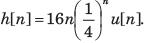So system is both stable and causal. ROC includes z = 1.

QUESTION: 3

### The transfer function of a system is given as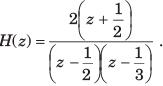Consider the two statements Statement(i) : System is causal and stable. Statement(ii) : Inverse system is causal and stable. The correct option is

Solution:

Pole of system at :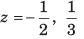Pole of inverse system at :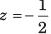For this system and inverse system all poles are inside |z|= 1. So both system are both causal and stable.

QUESTION: 4

The impulse response of a system is given by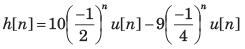For this system two statement are
Statement (i): System is causal and stable
Statement (ii): Inverse system is causal and stable.
The correct option is

Solution: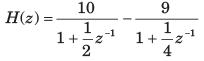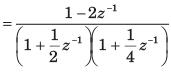Pole of this system are inside |z|= 1. So the system is stable and causal.
For the inverse system not all pole are inside |z|= 1.So inverse system is not stable and causal.

QUESTION: 5

The system
y[n] = cy[n - 1] -0.12 y[n - 2 ] + x[n - 1] + x[n - 2 ] is stable if

Solution: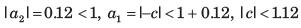QUESTION: 6

Consider the following three systems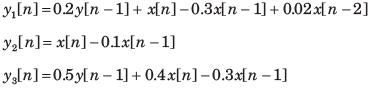The equivalent system are

Solution: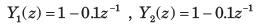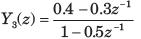So y1 and y2 are equivalent.

QUESTION: 7

The z-transform of a causal system is given as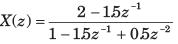The x is

Solution:

Causal signal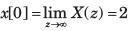QUESTION: 8

The z-transform of a anti causal system is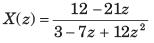The value of x is

Solution:

Anti causal signal,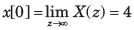QUESTION: 9

Given the z-transformsThe limit of x[∝] is

Solution:

The function has poles at z = 1, 3/4. Thus final value theorem applies.QUESTION: 10

The impulse response of the system shown in fig. P5.4.62 is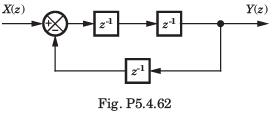Solution: